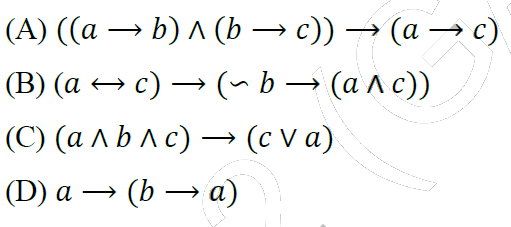Related Articles
GATE | GATE-CS-2014-(Set-2) | Question 63
• Difficulty Level : Basic
• Last Updated : 20 Mar, 2018

Which one of the following Boolean expressions is NOT a tautology?(A) A
(B) B
(C) C
(D) D

Answer: (B)

Explanation:
(A) a -> b means if ‘a’ is true then ‘b’ is also true.
Now, ‘b’ is true. Therefore, ‘c’ is also true.
=> Using transitivity rule , a -> c

(B) a <-> c is true if both ‘a’ and ‘c’ have same values.
Let ‘a’, ‘b’ and c’ be false.
Expression ‘a and b‘ is false and expression ‘not b’ is true.
RHS of the given equation should be true. But it evaluates to be false. Therefore, contradiction is there.

Option (B) is not a tautology.

(C) Let ‘a’ and ‘c’ be false. ’b’ be true
a and b and c is false
c or a is false

(D) Let ‘b’ be true.
b -> a means if ‘b’ is true then ‘a’ is also true.
Therefore, ‘a’ and ‘b’ both evaluates to be true.

Thus, option (B) is correct.

Please comment below if you find anything wrong in the above post.My Personal Notes arrow_drop_up